Home > A2C > Chapter 11 > Lesson 11.3.2 > Problem11-146

11-146.
1. For each equation, state the amplitude, period, vertical shift, horizontal shift, and sketch two cycles of the graph. Homework Help ✎

1. y = 3cos(2x)

2.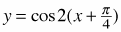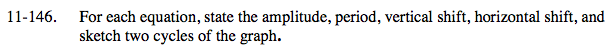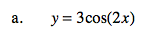The functions have the form of
y = a cos(b(xh)) + k.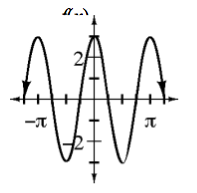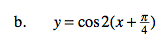Use 5 points to sketch one cycle and then repeat the pattern.
amplitude = 1
period = π

$\text{horizontal shift}=-\frac{\pi}{4}$